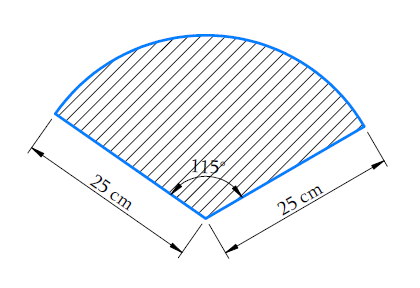# Ex.12.2 Q11 Areas Related to Circles Solution - NCERT Maths Class 10

Go back to  'Ex.12.2'

## Question

A car has two wipers which do not overlap. Each wiper has a blade of length $$\text{25 cm}$$ sweeping through an angle of $$115^\circ.$$Find the total area cleaned at each sweep of the blades.

## Text Solution

What is known?

A car has $$2$$ wipers which do not overlap. Each wiper has a blade length $$= 25 \,\rm{cm}$$ and sweeps through an angle $$\left( \theta \right) = {150^\circ}$$

What is unknown?

Total area cleaned at the sweep of the blade of the $$2$$ wipers.

Reasoning:Visually it is clear that

Area cleaned at the sweep of blades of each wiper $$=$$ Area of the sector with angle  $$115^\circ$$at the centre and radius of the circle $$\text{25 cm}$$

Since there are $$2$$ wipers of same blade length and same angle of sweeping. Also there is no area of overlap for the wipers.

$$\therefore \;$$Total area cleaned at each sweep of the blades $$=$$ \begin{align}2\ \times\end{align} Area cleaned at the sweep of each wiper.

Steps:

Area cleaned at the sweep of blades of each wiper = Area of the sector of a circle with radius $$\text {25 cm}$$ and of angle \begin{align}115^{\circ}\end{align}

\begin{align}& = \frac{\theta }{{{{360}^\circ }}} \times \pi {r^2}\\& = \frac{{{{115}^\circ }}}{{{{360}^\circ }}} \times \pi \times 25 \times 25\\& = \frac{{23}}{{72}} \times 625\pi \end{align}

Since there are $$2$$ identical blade length wipers

$$\therefore\;$$Total area cleaned at each sweep of the blades

\begin{align}& = 2 \times \frac{{23}}{{72}} \times 625\pi\\ & = 2 \times \frac{{23}}{{72}} \times \frac{{22}}{7} \times 625\\& = \frac{{23 \times 11 \times 625}}{{18 \times 7}}\\ &= \frac{{158125}}{{126}}\,{\text{c}}{{\text{m}}^{\text{2}}}\\ & = 1254.96\,\,\,{\text{c}}{{\text{m}}^{\text{2}}}\end{align}

Learn from the best math teachers and top your exams

• Live one on one classroom and doubt clearing
• Practice worksheets in and after class for conceptual clarity
• Personalized curriculum to keep up with school